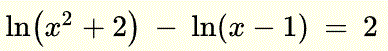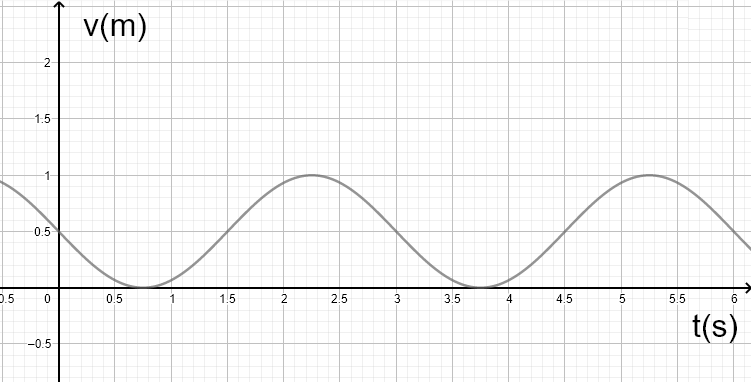# Grade 12 Math Practice Test

Grade 12 math practice test questions are presented along with their solutions on videos.

1. Solve the inequalityand present the solution set using intervals, number line and inequality symbols.
Solution on video at rational inequality, question 1

2. Solve the equationSolution on video at trigonometric equation, question 2

3. Solve the equationSolution on video at equation with logarithms, question 3

4. Solve the equationSolution on video at equation with exponentials, question 4

5. Verify the identity :Solution on video at verify trigonometric identities, question 5



6. Find the exact value of : $\displaystyle \quad \tan \left(\frac{13\pi}{12}\right)$
Solution on video at find exact value of trigonometric function, question 6

7. When polynomial $P(x)$ is divided by $x + 1$, the remainder is equal to $4$ and when $P(x)$ is divided by $x - 2$ it gives a remainder equal to $4$. Polynomial $p(x)$ has degree $3$ and has $x - 1$ as a factor. The leading coefficient of $P(x)$ is equal to $1$. Find $P(x)$
Solution on video at find polynomial given remainders and a factor, question 7

8. Function $f$ is defined by $f(x) = - x^4 - 5x^3 - 3x^2+9x$
a) Factor $f(x)$ completely.
b) Use the zeros to sketch the graph of $f$.
Solution on video at Factor completeley and sketch a polynomial, question 8

9. Find the equation of the polynomial function $g$ whose degree is equal to $4$ and whose graph is shown below and touches (does not cut) the x-axis at $x = -1$.Solution on video at Find the equation of a polynomial given its graph, question 9

10. For the function $y = - 0.5 \sin \left( 4(x+\frac{\pi}{16}) \right) + 2.5$, make a table of values over 1 period and sketch the graph over 2 periods.
Solution on video at make a table of values and sketch , question 10

11. The velocity $V$ in meters ( $m$ ) of an object is given by the graph below. Write $V$ as a function of time $t$ in seconds ( $s$ ) as a cosine function.Solution on video at find an equation to a trigonometric equation given by its graph , question 11

12. Given the function $y = \frac{2 x - 4}{x+2}$
a) Find the domain of the function
b) Find the x and y intercepts of the graph of the function
c) Find the equations of all the asymptotes of the function and any intercepts with the graph of the function
d) Make a table of signs and sketch the graph of the function
Solution on video at sketch the graph of the rational function y = (2x - 4) / (x + 2) , question 12

13. Given the function $y = \frac{x^2-9}{x+2}$
a) Find the domain of the function
b) Find the x and y intercepts of the graph of the function
c) Find the equations of all the asymptotes of the function
d) Make a table of signs and sketch the graph of the function

14. Find the equation of the rational function $h(x)$ whose graph is shown below and whose denominator has a polynomial of degree 2.AN: (2x-4)/[(x-1)(x-2)] with hole

15. Given the function $f(x) = -0.5 \log_2(x^2 - 1)-1$
a) Find the domain of the function
b) Find the x and y intercepts, if any, of the graph of the function
c) Find the equations of all the asymptotes, if any, of the function
d) Make a table of values and sketch the graph of the function

16. Given the function $h(x) = 2 + e^{(x-2)}$
a) Find the domain of the function
b) Find the x and y intercepts, if any, of the graph of the function
c) Find the equations of all the asymptotes, if any, of the function
d) Make a table of values and sketch the graph of the function

17. Given the function $h(x) = \ln (2x - 1) + 2$
a) Find the domain and range of function $h$.
b) Find the inverse of function $h$ and specify its domain and range.